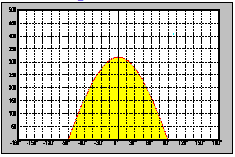The luminous intensity distribution pattern for a light source shows in which direction and with what intensity the light is emitted. The individual values can be presented in tabular or graphic form, and a number of diagrams are normally employed for this purpose. Luminous intensity is usually based on a 1000 lm light source.

C Plane SystemThe interior around the light source is treated as comprising a large number of planes, which can be divided up into the A, B and C plane systems. The most frequent procedure is to use the C plane system, with the common axis represented by a vertical line passing through the centre of the luminaire. Every plane is then defined in terms of the angle formed with a defined line of reference, e.g. the axis of the luminaire. Luminous intensity is usually measured in the C-plane at 15° intervals and for emission angles in 5° increments.

Polar CurveA polar curve  (luminous intensity distribution curve) normally shows the values measured in the C0/C180 and C90/C270 planes (transverse to and along the luminaire axis). For a rotationally symmetrical luminous intensity distribution pattern it is enough to show any one plane. The centre of the diagram corresponds to the midpoint of the luminaire.

Linear DiagramFor a linear diagram (Cartesian diagram) the emission angles are plotted along a straight x-axis and luminous intensity on the y-axis. Apart from that the values in the linear diagram correspond to those of the polar curve.